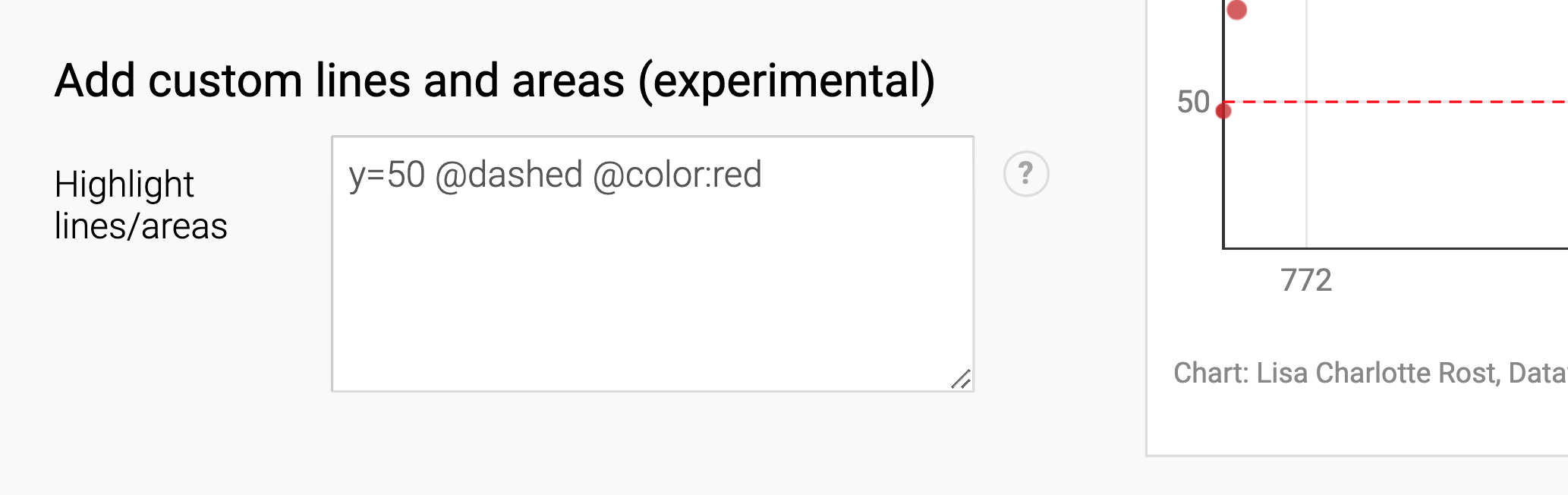# How to add custom lines and areas in scatterplots

In the last panel of the  Annotate tab in step 3: Visualize, we can add extra lines and areas to our scatterplots. That's helpful if we want to add a rectangle around/behind certain data dots, or if we want to show a threshold line.

For example, writing `y=50` will create a horizontal line at the vertical axis point "50 years" (the same works for the horizontal axis, e.g. `x=2000`):You can also define points, consisting of an x value and a y value, e.g. "2000, 50". If you define two points, a line will go from the first point to the second, e.g. "2000, 50, 4000, 70". Three or more points will define areas.

## Styling

We can design these lines and areas. Here are all options that are available:

 @dashed Makes a dashed line @dotted Makes a dotted line @color:red / @color:#cc0000 Colors the line/area @opacity:0.5 Makes the line/area more transparent (values between 0 and 1 are possible) @stroke:red / @stroke:#cc0000 Creates and colors the stroke around an area @width:2 Defines the width of the stroke around an area

## Try it out!

Here are examples you can copy & paste in the field. You might need to adjust the numbers, since your horizontal axis and vertical axis range might differ from the one in which we tried

 y = 50 Created a horizontal line that goes all the way through the chart on the height of y = 50. x= 50 Created a vertical line that goes all the way through the chart on the height of x = 50. 2000, 50, 4000, 70 Creates a line that goes from the point 2000,50 to the point 4000, 70. 0,0,0,5,5,5,5,0 @color:red @opacity:0.5 Creates a square, then set the color to red and make it half-transparent. y = sin(x*20) @color:#1E4363  @width:2 Creates a sinus curve, make it blue and make the line a bit thicker. Functions like cos(x) and tan(x) are also supported.

💡 When working with data in date format, drawing lines by writing an equation such as "y = mx + c" doesn't work. Instead, you can draw a line by defining two points. For example, if you want to draw a straight line between (x, y) = (August 25 2020, 50) and (July 5 2020, 100), you can write: `25/08/2020, 50, 05/06/2020, 100 @color:gray`